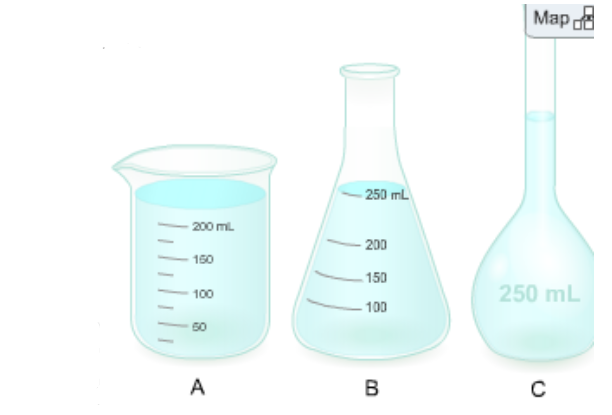# Problem: You need to prepare a 250. mL of a 0.850 M aqueous solution of sucrose, C12H22O11(aq), which is used frequently in biological experiments.Which type of glassware should you use to make this solution (assuming that the accuracy of the concentration is important)?A. AB. BC. CHow should the correct amount of solute be obtained?A. Measure out x mol of solid sucrose on a molemeter.B. Measure out x cm of sucrose with a ruler.C. Measure out x g of solid sucrose on a balance.Now that you have selected the correct unit above, what is the value of x?

###### FREE Expert Solution

In preparing solutions, where great accuracy of concentration is required, a volumetric flask is used.

98% (377 ratings)###### Problem Details

You need to prepare a 250. mL of a 0.850 M aqueous solution of sucrose, C12H22O11(aq), which is used frequently in biological experiments.

Which type of glassware should you use to make this solution (assuming that the accuracy of the concentration is important)?A. A
B. B
C. C

How should the correct amount of solute be obtained?

A. Measure out x mol of solid sucrose on a molemeter.
B. Measure out x cm of sucrose with a ruler.
C. Measure out x g of solid sucrose on a balance.

Now that you have selected the correct unit above, what is the value of x?